Win up to 100% scholarship on Aakash BYJU'S JEE/NEET courses with ABNAT Win up to 100% scholarship on Aakash BYJU'S JEE/NEET courses with ABNAT

# JEE Main 2022 July 26 – Shift 1 Physics Question Paper with Solutions

The JEE Main 2022 July 26 – Shift 1 Physics Question Paper with Solutions is given on this page. Students can understand the marks distribution and difficulty level by checking JEE Main 2022 question papers. They can use JEE Main 2022 answer keys to find out their expected scores. They are advised to refer to the JEE Main question papers. Solving the JEE Main 2022 question paper with solutions will definitely take students’ JEE Main preparation to the next level.

## JEE Main 2022 July 26th Shift 1 Physics Question Paper and Solutions

SECTION – A

Multiple Choice Questions: This section contains 20 multiple choice questions. Each question has 4 choices (1), (2), (3) and (4), out of which ONLY ONE is correct.

1. Three masses M = 100 kg, m1 = 10 kg and m2 = 20 kg are arranged in a system as shown in figure. All the surfaces are frictionless and strings are inextensible and weightless. The pulleys are also weightless and frictionless. A force F is applied on the system so that the mass m2 moves upward with an acceleration of 2 ms–2. The value of F is

(Take g = 10 ms–2)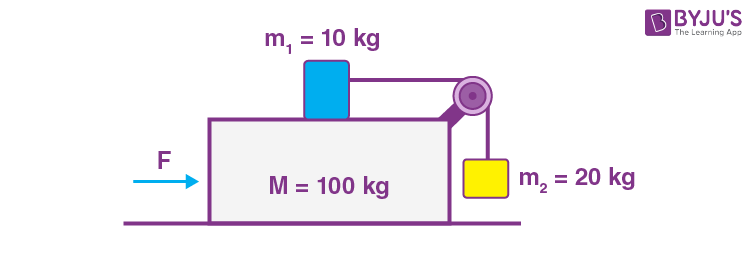(A) 3360 N

(B) 3380 N

(C) 3120 N

(D) 3240 N

Sol. In frame of block of mass M movingwithacceleration a.m1aT = 2m1⇒ 10aT = 20 …(i)Tm2g = m22 ⇒T – 200 = 40 ⇒T = 240 …(ii)

⇒ From equation 1 and 2 10a = 260 or a = 26 m/s2

for block

F = (M + m2)a = 120 × 26

= 3120 N

2. A radio can tune to any station in 6 MHz to 10 MHz band. The value of corresponding wavelength bandwidth will be

(A) 4 m

(B) 20 m

(C) 30 m

(D) 50 m

Sol.

$$\begin{array}{l}\nu_1 = 6 \times 10^6~\text{Hz}\\ \Rightarrow \lambda_1=\frac{3\times10^8}{6\times10^6}=50\text{ m} \end{array}$$
$$\begin{array}{l}\nu_2 = 10 \times 10^6~\text{Hz} \Rightarrow \lambda_2=\frac{3\times10^8}{10\times10^6}=30\text{ m}\end{array}$$
$$\begin{array}{l}\Rightarrow \text{Wavelength band with =}\left|\lambda_1-\lambda_2\right|= 20\text{m} \end{array}$$

3. The disintegration rate of a certain radioactive sample at any instant is 4250 disintegrations per minute. 10 minutes later, the rate becomes 2250 disintegrations per minute. The approximate decay constant is

(Take log101.88 = 0.274)

(A) 0.02 min–1

(B) 2.7 min–1

(C) 0.063 min–1

(D) 6.3 min–1

Sol.

$$\begin{array}{l}A_0 = 4250\\ A = 2250 = A_0e^{-\lambda t}\end{array}$$
$$\begin{array}{l} \Rightarrow \frac{2250}{4250}=e^{-\lambda t}\end{array}$$
$$\begin{array}{l} \Rightarrow \lambda\left(10\right)=\text{In }\left(\frac{4250}{2250}\right)\end{array}$$
$$\begin{array}{l}\lambda(10) = 0.636\\ \lambda = 0.063\end{array}$$

4. A parallel beam of light of wavelength 900 nm and intensity 100 Wm–2 is incident on a surface perpendicular to the beam. The number of photons crossing 1 cm–2 area perpendicular to the beam in one second is

(A) 3 × 1016

(B) 4.5 × 1016

(C) 4.5 × 1017

(D) 4.5 × 1020

Sol.

$$\begin{array}{l} \lambda = 900 ~nm\\ I = 100 ~\frac{W}{m^2}\\ A = 10^{-4}\\ \Rightarrow P = 10^{-2}~ W\\ \Rightarrow \text{Number of photons incident per second} \end{array}$$
$$\begin{array}{l} =\frac{10^{-2}\lambda}{hc} \end{array}$$
$$\begin{array}{l} =\frac{9\times10^{-11}\times10^2}{6.63\times10^{-34}\times 3\times10^8}\simeq 4.5\times10^{16}\end{array}$$

5. In Young’s double slit experiment, the fringe width is 12 mm. If the entire arrangement is placed in water of refractive index 4/3, then the fringe width becomes (in mm)

(A) 16

(B) 9

(C) 48

(D) 12

Sol.

B = 12 × 10-3

$$\begin{array}{l}\beta’=\frac{\beta}{\mu}=\frac{12\times 10^{-3}}{\frac{4}{3}}\end{array}$$

= 9 × 10-3 m = 9 mm

6. The magnetic field of a plane electromagnetic wave is given by

$$\begin{array}{l} \overrightarrow{B}=2\times10^{-8}\sin\left(0.5\times10^3x+1.5\times10^{11}t\right) \hat{j}T.\end{array}$$

The amplitude of the electric field would be

(A) 6 Vm–1 along x-axis

(B) 3 Vm–1 along z-axis

(C) 6 Vm–1 along z-axis

(D) 2 × 10–8Vm–1 along z-axis

Sol.

$$\begin{array}{l}\text{Speed of light c }=\frac{\omega}{k}=\frac{1.5\times10^{11}}{0.5\times10^3}=3\times10^8\textup{ m/sec}\end{array}$$
$$\begin{array}{l}So, E_0 = B_0c\\ = 2 \times 10^{-8} \times 3 \times 10^8 = 6\ \text{V/m}\end{array}$$

Direction will be along z-axis.

7. In a series LR circuit XL = R and power factor of the circuit is P1. When capacitor with capacitance C such that XL = XC is put in series, the power factor becomes P2. The ratio P1/P2 is

$$\begin{array}{l} (\text{A})\ \frac{1}{2}\end{array}$$
$$\begin{array}{l} (\text{B})\ \frac{1}{\sqrt{2}}\end{array}$$
$$\begin{array}{l} (\text{C})\ \frac{\sqrt{3}}{\sqrt{2}}\end{array}$$
$$\begin{array}{l}\left(D\right) 2~\colon 1\end{array}$$

Sol.

$$\begin{array}{l} P_1=\cos\phi=\frac{1}{\sqrt{2}}\left(X_L=R\right) \end{array}$$
$$\begin{array}{l}P_2 = cos\phi ‘ = 1 \left(\text{will become resonance circuit}\right)\end{array}$$

So,

$$\begin{array}{l} \frac{P_1}{P_2}=\frac{1}{\sqrt{2}} \end{array}$$

8. A charge particle is moving in a uniform field

$$\begin{array}{l} \left(2\hat{i}+3\hat{j}\right)T.\ \text{If it has an acceleration of }\ \left(\alpha\hat{i}-4\hat{j}\right)\text{m/s}^2,\end{array}$$
then the value of α will be

(A) 3

(B) 6

(C) 12

(D) 2

As magnetic force is perpendicular to magnetic field So,

$$\begin{array}{l} \overrightarrow{F}\cdot\overrightarrow{B}\end{array}$$
must be 0

$$\begin{array}{l}\text{So,} ~2\alpha – 12 = 0 \\ \alpha = 6\end{array}$$

9. BX and BY are the magnetic field at the centre of two coils X and Y, respectively each carrying equal current. If coil X has 200 turns and 20 cm radius and coil Y has 400 turns and 20 cm radius, the ratio of BX and BY is

(A) 1 : 1

(B) 1 : 2

(C) 2 : 1

(D) 4 : 1

Sol.

$$\begin{array}{l} B=\frac{\mu_0NI}{2R}\end{array}$$
$$\begin{array}{l} \frac{B_x}{B_y}=\frac{N_xR_y}{N_yR_x} \end{array}$$
$$\begin{array}{l} =\frac{200\times20}{400\times20}=\frac{1}{2} \end{array}$$

10. The current I in the given circuit will be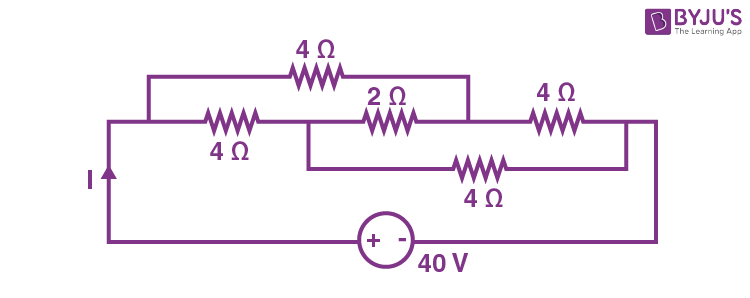(A) 10 A

(B) 20 A

(C) 4 A

(D) 40 A

Sol.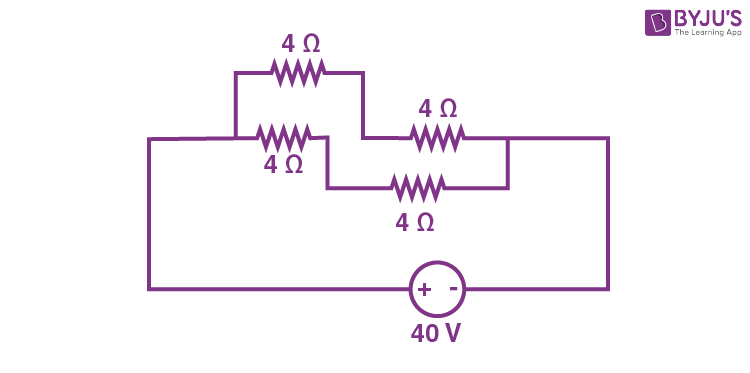The grouping of resistance is a wheatstone bridge.

So, Rnet = 4 Ω

So,

$$\begin{array}{l}i=\frac{V}{R_{\text{net}}}=10\ \text{A}\end{array}$$

11. The total charge on the system of capacitors

$$\begin{array}{l}C_1 = 1 \mu\text{F,} C_2 = 2 \mu\text{F,} C_3 = 4 \mu\text{F}~\text{and}~ C_4 = 3 \mu\text{F,}\end{array}$$
connected in parallel is :

(Assume a battery of 20 V is connected to the combination)

(A) 200 μC

(B) 200 C

(C) 10 μC

(D) 10 C

Sol. Equivalent C = ΣCi

= 10 μF

⇒ Charge Q = CV

= 200 μC

12. When a particle executes Simple Harmonic Motion, the nature of graph of velocity as a function of displacement will be :

(A) Circular

(B) Elliptical

(C) Sinusoidal

(D) Straight line

Sol.

$$\begin{array}{l}Let~ x = Asin \omega t \\ \Rightarrow v = A\omega cos\omega t \end{array}$$
$$\begin{array}{l} \Rightarrow v=\pm\omega\sqrt{A^2-x^2}\end{array}$$
$$\begin{array}{l} \Rightarrow \frac{v^2}{\omega^2}+x^2=A^2\end{array}$$

Ellipse

13. 7 mol of a certain monoatomic ideal gas undergoes a temperature increase of 40 K at constant pressure. The increase in the internal energy of the gas in this process is :

(Given R = 8.3 JK–1 mol–1)

(A) 5810 J

(B) 3486 J

(C) 11620 J

(D) 6972 J

Sol.

$$\begin{array}{l}\triangle U = nC_v\triangle T =7\times\frac{3R}{2}\times 40 = 3486 ~\text{J}\end{array}$$

14. A monoatomic gas at pressure P and volume V is suddenly compressed to one eighth of its original volume. The final pressure at constant entropy will be :

(A) P

(B) 8P

(C) 32P

(D) 64P

Sol.

$$\begin{array}{l} PV^\gamma = constant\end{array}$$
$$\begin{array}{l} \Rightarrow PV^\gamma=\left(P’\right)\left(\frac{v}{8}\right)^\gamma\text{ where }\gamma = 5/3 \\\Rightarrow P’= 32P \end{array}$$

15. A water drop of radius 1 cm is broken into 729 equal droplets. If surface tension of water is 75 dyne/cm, then the gain in surface energy upto first decimal place will be :

(Given π = 3.14)

(A) 8.5 × 10–4 J

(B) 8.2 × 10–4 J

(C) 7.5 × 10–4 J

(D) 5.3 × 10–4 J

Sol.

$$\begin{array}{l} 729\times\frac{4}{3}\pi r^3=\frac{4}{3}\pi R^3 \end{array}$$

⇒ R = 9r ……(1)

ΔU = S × ΔA …..(2)

$$\begin{array}{l}\Rightarrow \triangle U = S \times \{-4 \pi R^2 + 729 \times 4 \pi r^2\} \end{array}$$
$$\begin{array}{l}= S \times 4\pi\{729r^2 – 81r^2\}\\ = 7.5 \times 10^{-4}\text{ J}\end{array}$$

16. The percentage decrease in the weight of a rocket, when taken to a height of 32 km above the surface of earth will, be:

(Radius of earth = 6400 km)

(A) 1%

(B) 3%

(C) 4%

(D) 0.5%

Sol.

$$\begin{array}{l} \because\ g=\frac{GM}{r^2} \end{array}$$
$$\begin{array}{l} \Rightarrow \frac{\Delta g}{g}=2\frac{\Delta r}{r}\end{array}$$
$$\begin{array}{l} \Rightarrow \frac{\Delta g}{g}\times 100=2\times\frac{32}{6400}\times100\%=1\% \end{array}$$

⇒ % decrease in weight = 1%

17. As per the given figure, two blocks each of mass 250 g are connected to a spring of spring constant 2 Nm–1. If both are given velocity v in opposite directions, then maximum elongation of the spring is:$$\begin{array}{l} (\text{A})\ \frac{v}{2\sqrt{2}}\end{array}$$
$$\begin{array}{l} (\text{B})\ \frac{v}{2}\end{array}$$
$$\begin{array}{l} (\text{C})\ \frac{v}{4}\end{array}$$
$$\begin{array}{l} (\text{D})\ \frac{v}{\sqrt{2}}\end{array}$$

Sol. ∵ Loss in KE = Gain in spring energy

$$\begin{array}{l} \Rightarrow \frac{1}{2}mv^2\times2=\frac{1}{2}kx_m^2\end{array}$$
$$\begin{array}{l} \Rightarrow 2\times\frac{1}{4}\times v^2=2\times x_m^2\end{array}$$
$$\begin{array}{l} \Rightarrow x_m=\sqrt{\frac{v^2}{4}}=\frac{v}{2}\end{array}$$

18. A monkey of mass 50 kg climbs on a rope which can withstand the tension (T) of 350 N. If monkey initially climbs down with an acceleration of 4 m/s2 and then climbs up with an acceleration of 5 m/s2. Choose the correct option (g = 10 m/s2).

(A) T = 700 N while climbing upward

(B) T = 350 N while going downward

(C) Rope will break while climbing upward

(D) Rope will break while going downward

Sol. Tdown = 50 × (10 – 4)

= 50 × 6

= 300 N

Tup = 50 × (10 + 5)

= 50 × 15

= 750 N

⇒ Rope will break while climbing up.

19. Two projectiles thrown at 30° and 45° with the horizontal, respectively, reach the maximum height in same time. The ratio of their initial velocities is :

$$\begin{array}{l} (\text{A})\ 1:\sqrt{2} \end{array}$$
$$\begin{array}{l} (\text{B}) 2 ~\colon 1 \end{array}$$
$$\begin{array}{l} (\text{C})\ \sqrt{2}:1\end{array}$$
$$\begin{array}{l} (\text{D})\ 1~\colon 2 \end{array}$$

Sol.

$$\begin{array}{l} \because\ t_a=\frac{u\sin\theta}{g}\end{array}$$
$$\begin{array}{l} \Rightarrow \frac{u_1\sin\left(30^\circ\right)}{g}=\frac{u_2\sin\left(45^\circ\right)}{g}\end{array}$$
$$\begin{array}{l} \Rightarrow \frac{u_1}{u_2}=\frac{\frac{1}{\sqrt{2}}}{\frac{1}{2}}=\frac{\sqrt{2}}{1}\end{array}$$

20. A screw gauge of pitch 0.5 mm is used to measure the diameter of uniform wire of length 6.8 cm, the main scale reading is 1.5 mm and circular scale reading is 7. The calculated curved surface area of wire to appropriate significant figures is :

[Screw gauge has 50 divisions on its circular scale]

(A) 6.8 cm2

(B) 3.4 cm2

(C) 3.9 cm2

(D) 2.4 cm2

Sol.

$$\begin{array}{l} \text{Least count=}\frac{0.5}{50}\text{ mm}=0.01\text{ mm}\end{array}$$
$$\begin{array}{l}\therefore\text{Diameter, d =} 1.5~ \text{mm} + 7 \times 0.01\\ = 1.57 \text{mm}\end{array}$$
$$\begin{array}{l}\therefore \text{Surface area =} \left(2\pi r\right) \times l = \pi dl\end{array}$$
$$\begin{array}{l} =3.142\times\frac{1.57}{10}\times 6.8\ \text{cm}^2\\ = 3.354 ~\text{cm}^2 = 3.4 ~\text{cm}^2\end{array}$$

SECTION – B

Numerical Value Type Questions: This section contains 10 questions. In Section B, attempt any five questions out of 10. The answer to each question is a NUMERICAL VALUE. For each question, enter the correct numerical value (in decimal notation, truncated/rounded-off to the second decimal place; e.g. 06.25, 07.00, –00.33, –00.30, 30.27, –27.30) using the mouse and the on-screen virtual numeric keypad in the place designated to enter the answer.

1. If the initial velocity in horizontal direction of a projectile is unit vector

$$\begin{array}{l} \hat{i} \end{array}$$
and the equation of trajectory is y = 5x(1 – x). The y component vector of the initial velocity is ___
$$\begin{array}{l} \hat{j}.\end{array}$$

$$\begin{array}{l}\left(\text{Take}~ g = 10~m/s^2\right)\end{array}$$

Sol.

$$\begin{array}{l}y = 5x – 5x^2\\y = x tan \theta – \frac{1}{2}\frac{gx^2}{v^2} \end{array}$$
$$\begin{array}{l} \tan\theta = 5 =\frac{u_y}{u_x}\\\Rightarrow u_y= 5\end{array}$$

2. A disc of mass 1 kg and radius R is free to rotate about a horizontal axis passing through its centre and perpendicular to the plane of disc. A body of same mass as that of disc of fixed at the highest point of the disc. Now the system is released, when the body comes to the lowest position, it angular speed will be

$$\begin{array}{l} 4\sqrt{\frac{x}{3R}} ~\text{rad s}^{-1}\end{array}$$
where x = ___.

(g = 10 ms–2)

Sol.Loss in P.E = Gain in K.E.

$$\begin{array}{l} 2\text{mg}R=\frac{1}{2}\left[\frac{1}{2}mR^2+mR^2\right]w^2 \end{array}$$
$$\begin{array}{l} 2\text{mg}R=\frac{1}{2}\times\frac{3}{2}mR^2w^2\end{array}$$
$$\begin{array}{l} w^2=\frac{8g}{3R}\end{array}$$
$$\begin{array}{l} w=\sqrt{\frac{8g}{3R}}=4\sqrt{\frac{g}{2\times3R}}\end{array}$$
$$\begin{array}{l} \Rightarrow x=\frac{g}{2}=5\end{array}$$

3. In an experiment of determine the Young’s modulus of wire of a length exactly 1 m, the extension in the length of the wire is measured as 0.4 mm with an uncertainty of ±0.02 mm when a load of 1 kg is applied. The diameter of the wire is measured as 0.4 mm with an uncertainty of ±0.02 mm when a load of 1 kg is applied. The diameter of the wire is measured as 0.4 mm with an uncertainty of ±0.01 mm. The error in the measurement of Young’s modulus (ΔY) is found to be x × 1010 Nm–2. The value of x is ___.

$$\begin{array}{l}\left(\text{Take}~ g = 10~m/s^2\right)\end{array}$$

Sol.

$$\begin{array}{l} \frac{F/A}{l/L}=Y, A=\pi D^2\end{array}$$
$$\begin{array}{l} \frac{\Delta Y}{Y}=\frac{\Delta F}{F}+\frac{2\Delta D}{D}+\frac{\Delta l}{e}+\frac{\Delta L}{L}\end{array}$$
$$\begin{array}{l} =2\times\frac{0.01}{0.4}+\frac{0.02}{0.4} \end{array}$$
$$\begin{array}{l} =\frac{0.04}{0.4}=\frac{1}{10}\end{array}$$
$$\begin{array}{l} Y=\frac{Fl}{A\Delta l} \end{array}$$
$$\begin{array}{l} =\frac{10\times1}{\pi\left(0.1\text{ mm}\right)^2\times 0.4\text{ mm}}\\= 1.988 \times 10^{11}\\\approx 2 \times 10^{11} \end{array}$$
$$\begin{array}{l} \frac{\Delta y}{y}=\frac{1}{10}\end{array}$$
$$\begin{array}{l} \Delta y=\frac{y}{10}=2\times 10^{10} \end{array}$$

4. When a car is approaching the observer, the frequency of horn is 100 Hz. After passing the observer, it is 50 Hz. If the observer moves with the car, the frequency will be

$$\begin{array}{l} \frac{x}{3} \end{array}$$
Hz where x = ___.

Sol.

$$\begin{array}{l} 100=v_0\frac{v}{v-v_c}\end{array}$$
$$\begin{array}{l} 50=v_0\frac{v}{v+v_c}\end{array}$$
$$\begin{array}{l} 2=\frac{v+v_c}{v-v_c}\end{array}$$
$$\begin{array}{l}2v – 2v_c = v + v_c\\v_c=\frac{v}{3}\end{array}$$
$$\begin{array}{l} 100=v_0\frac{v\times3}{2v}\Rightarrow v_0=\frac{200}{3}=\frac{x}{3}\Rightarrow x = 200\end{array}$$

5. A composite parallel plate capacitor is made up of two different dielectric materials with different thickness (t1 and t2) as shown in figure. The two different dielectric materials are separated by a conducting foil F. The voltage of the conducting foil is ___V.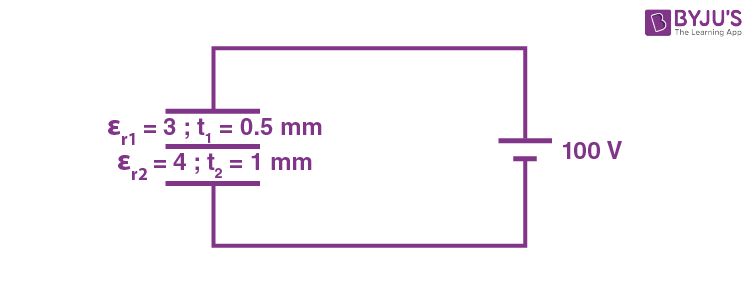Sol.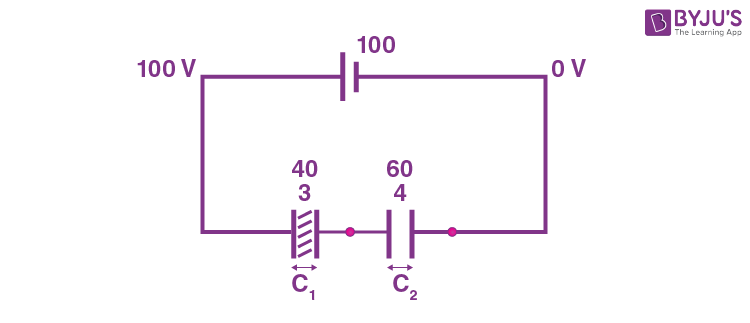$$\begin{array}{l} \frac{C_1}{C_2}=\frac{3\times t_2}{t_1\times 4}=\frac{3}{2}\end{array}$$
$$\begin{array}{l} \frac{q}{C_1}=v_1,\frac{q}{C_2}=v_2\end{array}$$
$$\begin{array}{l} \frac{v_1}{v_2}=\frac{C_2}{C_1}=\frac{2}{3} \end{array}$$

6. Resistances are connected in a meter bridge circuit as shown in the figure. The balancing length l1 is
40 cm. Now an unknown resistance x is connected in series with P and new balancing length is found to be 80 cm measured from the same end. Then the value of x will be ________ Ω.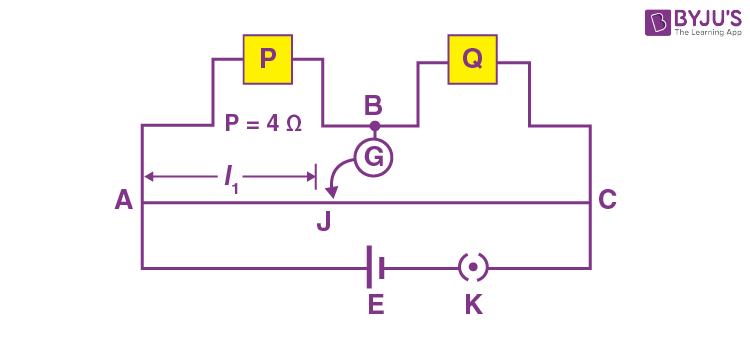Sol.

$$\begin{array}{l} \frac{P}{40}=\frac{Q}{60}\cdots\left(1\right)\end{array}$$
$$\begin{array}{l} \frac{P+x}{80}=\frac{Q}{20}\cdots\left(2\right)\end{array}$$
$$\begin{array}{l} \frac{P}{P+x}\times\frac{80}{40}=\frac{20}{60} \end{array}$$
$$\begin{array}{l} \frac{4}{4+x}\times 2=\frac{1}{3} \end{array}$$

24 = 4 + x

x = 20

7. The effective current I in the given circuit at very high frequencies will be ________ A.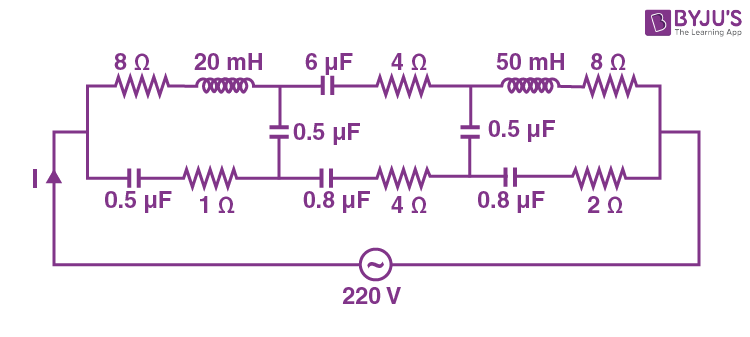Sol. Equivalent circuit will be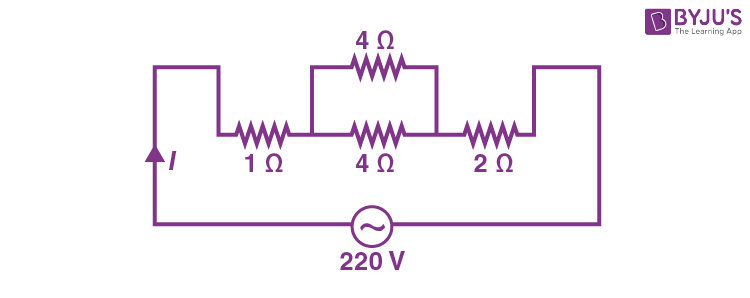$$\begin{array}{l} I=\frac{220}{5}=44\text{ A}\end{array}$$

8. The graph between 1/u and 1/v for a thin convex lens in order to determine its focal length is plotted as shown in the figure. The refractive index of lens is 1.5, and its both the surfaces have the same radius of curvature R. The value of R will be______ cm.

(where u = object distance,v = image distance)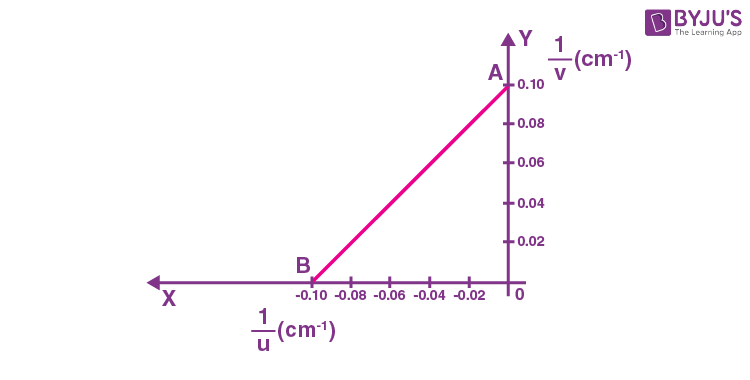Sol.

$$\begin{array}{l}f = 10 \text{cm} \\\frac{1}{f}=\left(\mu-1\right)\left(\frac{1}{R}-\frac{1}{-R}\right)\end{array}$$
$$\begin{array}{l} \frac{1}{10}=\frac{1.5-1}{1}\times \frac{2}{R}\end{array}$$
$$\begin{array}{l} \frac{1}{10}=\frac{1}{R}\\R = 10~ \text{cm}\end{array}$$

9. In the hydrogen spectrum, λ be the wavelength of first transition line of Lyman series. The wavelength difference will be “aλ” between the wavelength of 3rd transition line of the Paschen series and that of 2nd transition line of Balmer series where a = _______.

Sol.

$$\begin{array}{l} \frac{1}{\lambda}=R_H\left(\frac{1}{1^2}-\frac{1}{2^2}\right)\end{array}$$
$$\begin{array}{l} \frac{1}{\lambda_3}=R_H\left(\frac{1}{3^2}-\frac{1}{6^2}\right)\end{array}$$
$$\begin{array}{l} \frac{1}{\lambda_2}=R_H\left(\frac{1}{2^2}-\frac{1}{4^2}\right) \end{array}$$
$$\begin{array}{l}\therefore \lambda_3 – \lambda_2 = a\lambda\\a = 5\end{array}$$

10. In the circuit shown below, maximum Zener diode current will be ________ mA.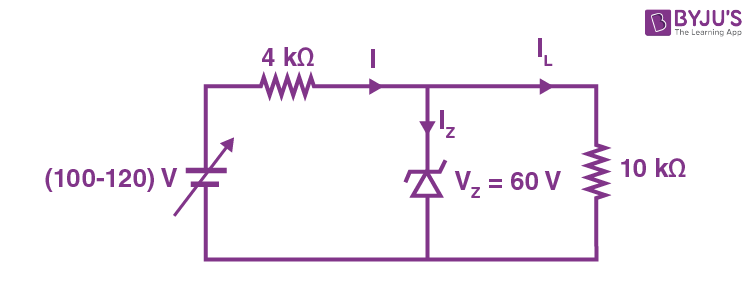Sol.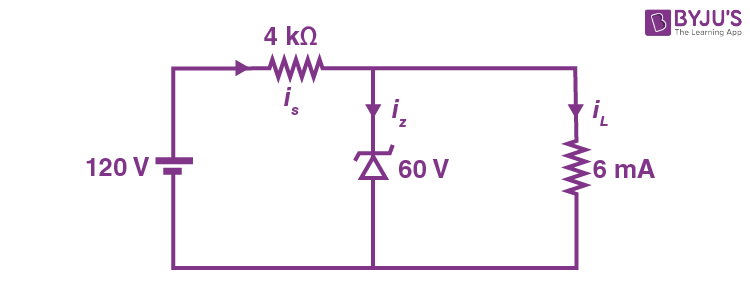$$\begin{array}{l} i_s=\frac{60}{4\times10^3}=15\times10^{-3}=15\text{ mA}\end{array}$$
$$\begin{array}{l}i_L=\frac{60}{10\times10^3}=6\text{ mA}\end{array}$$
$$\begin{array}{l}I_z = i_s – i_L = 9~ \text{mA}\end{array}$$

### Download PDF of JEE Main 2022 July 26 Shift 1 Physics Paper & Solutions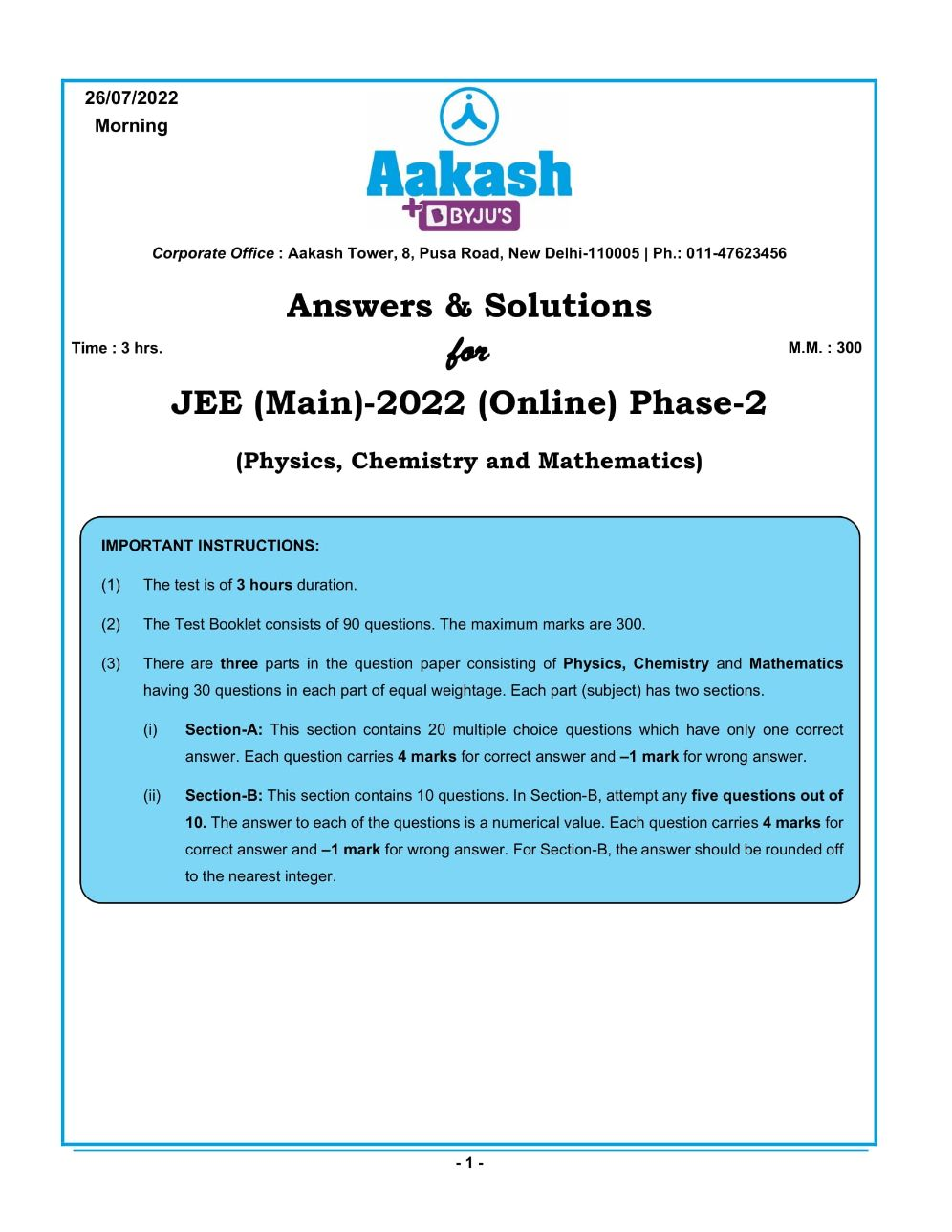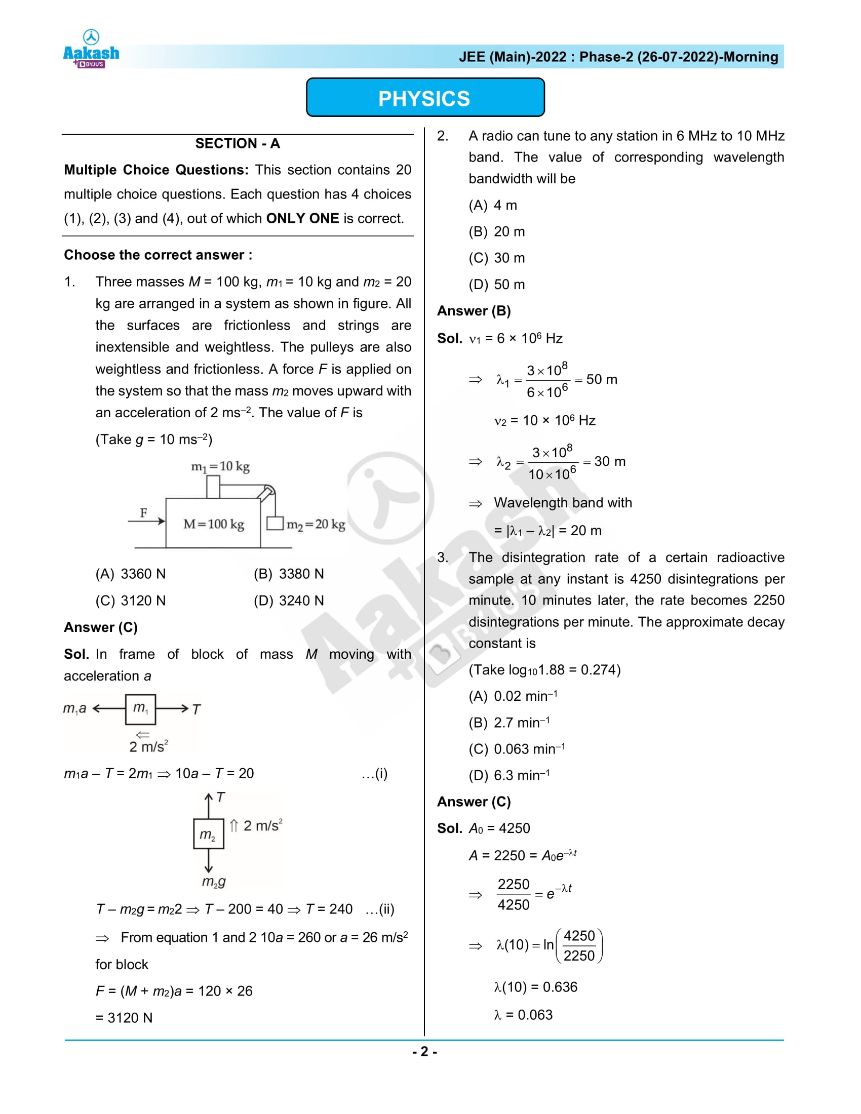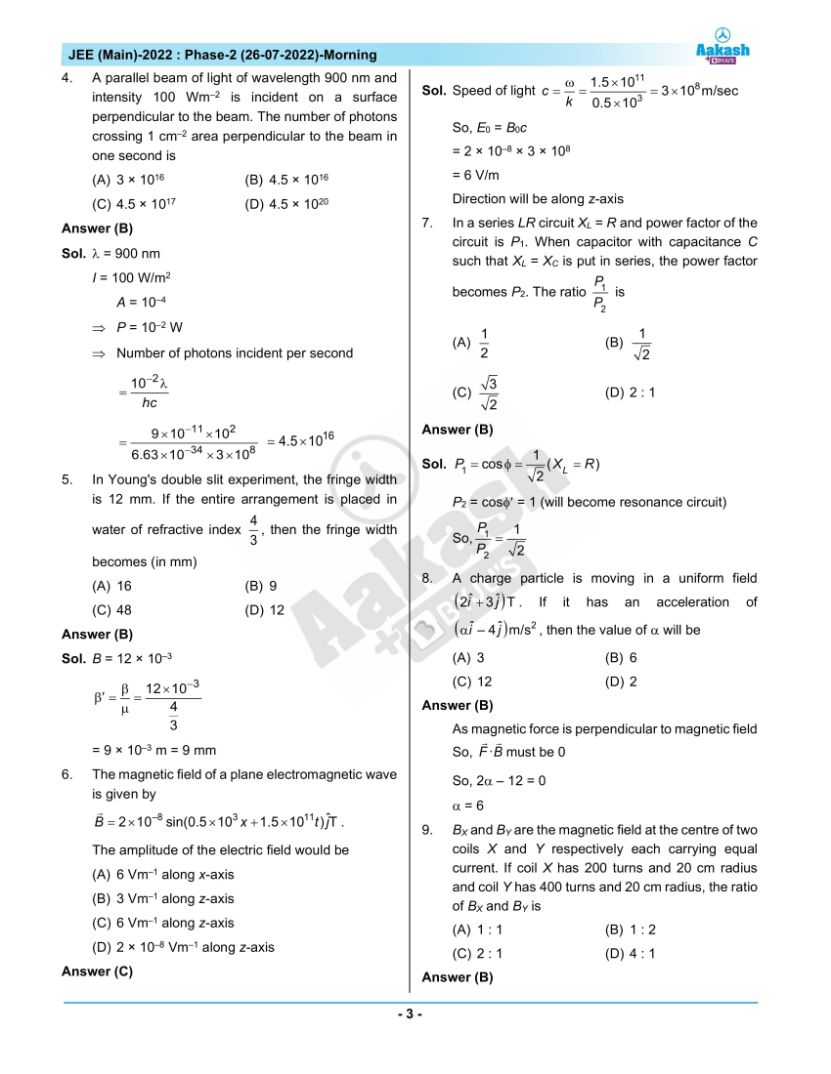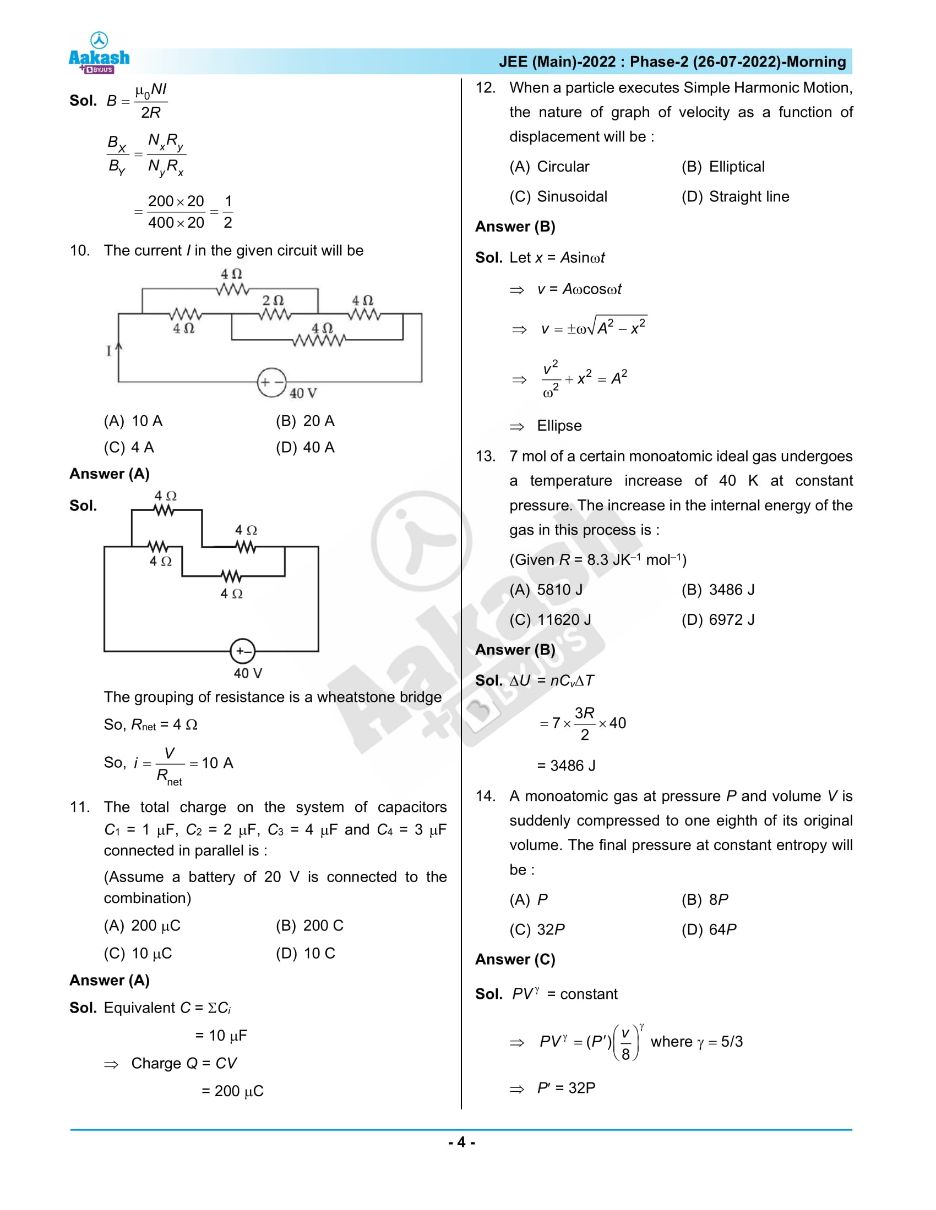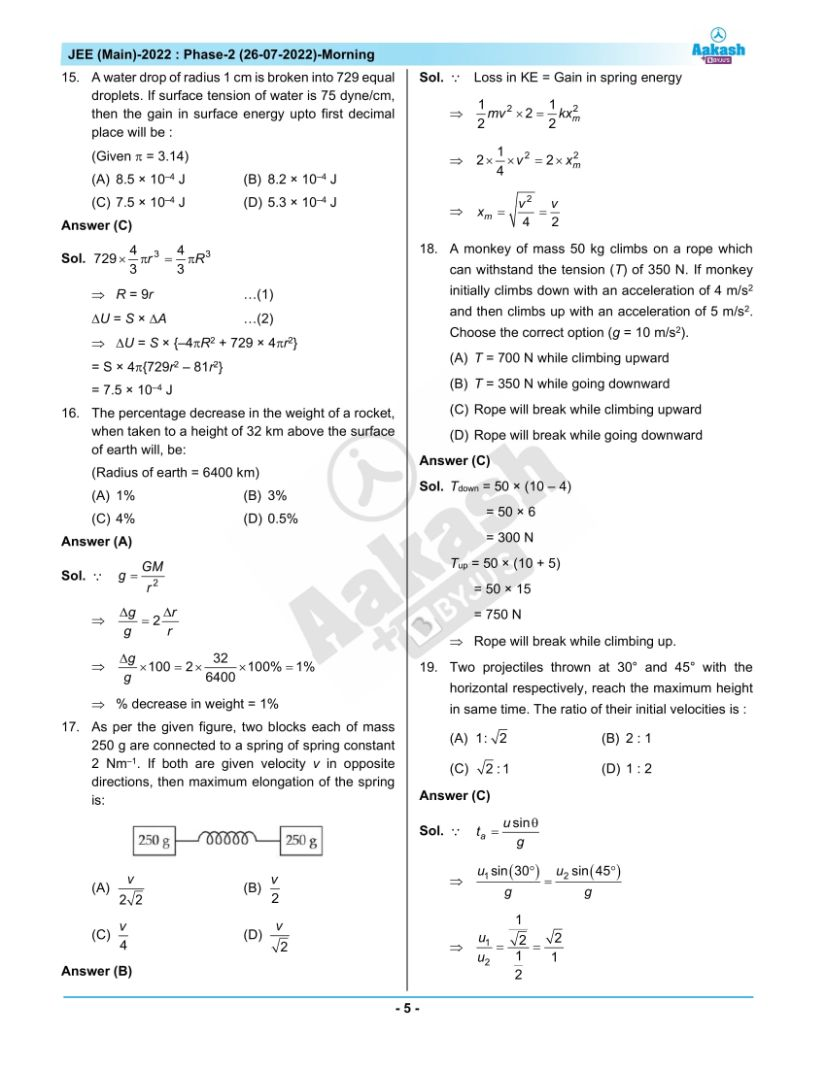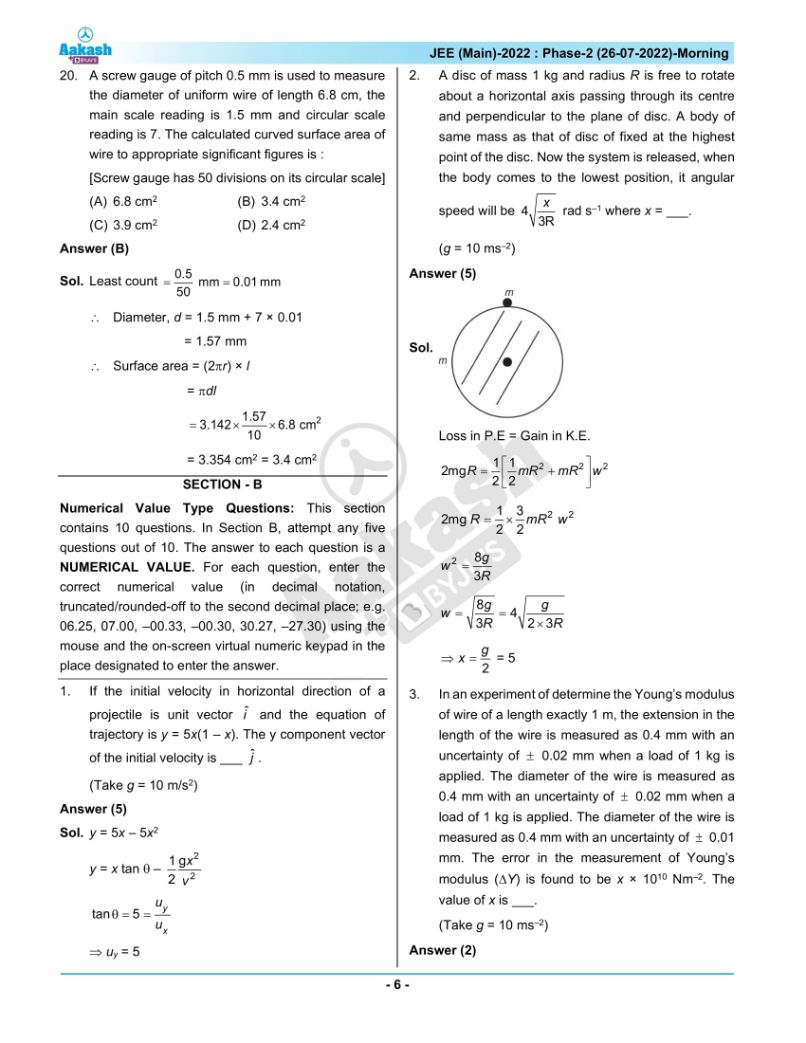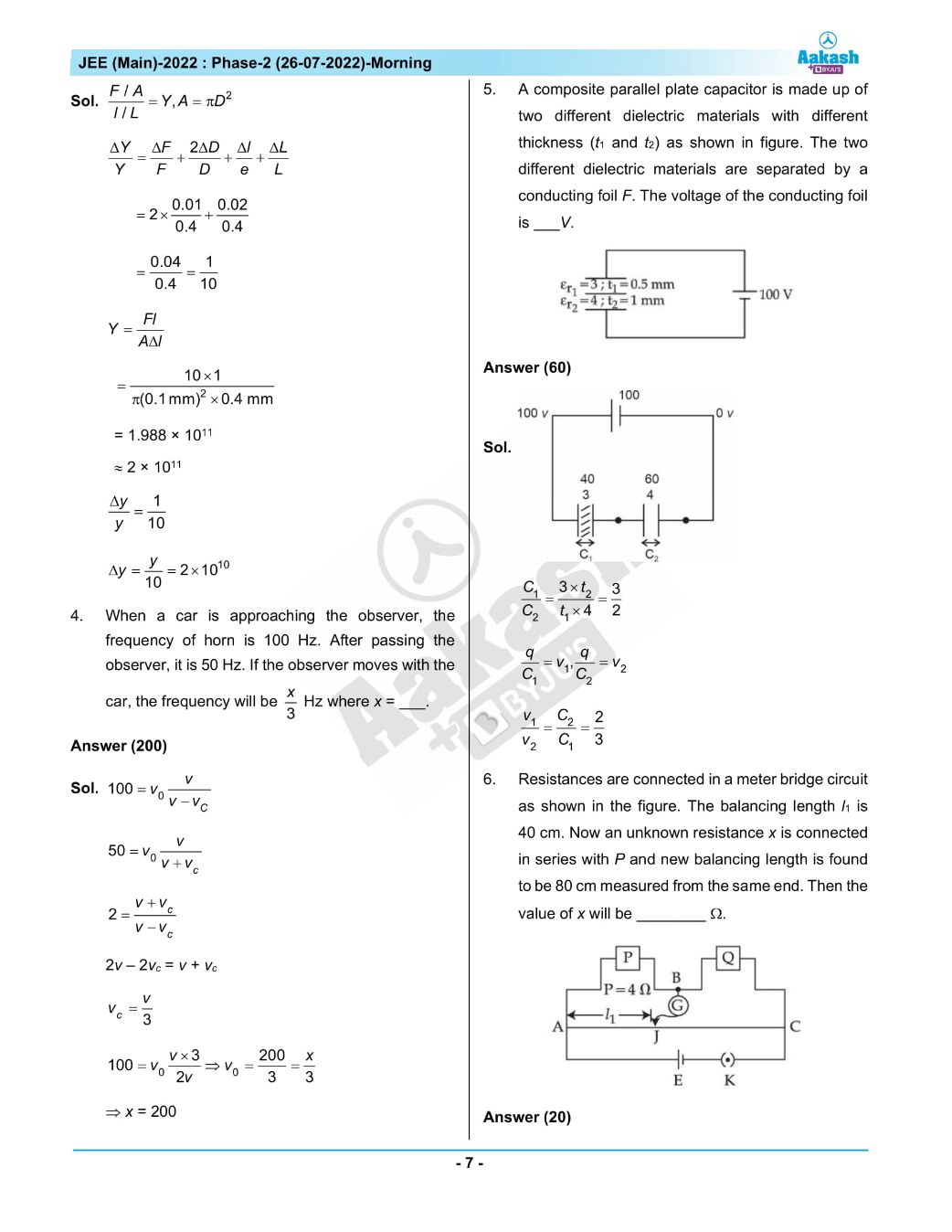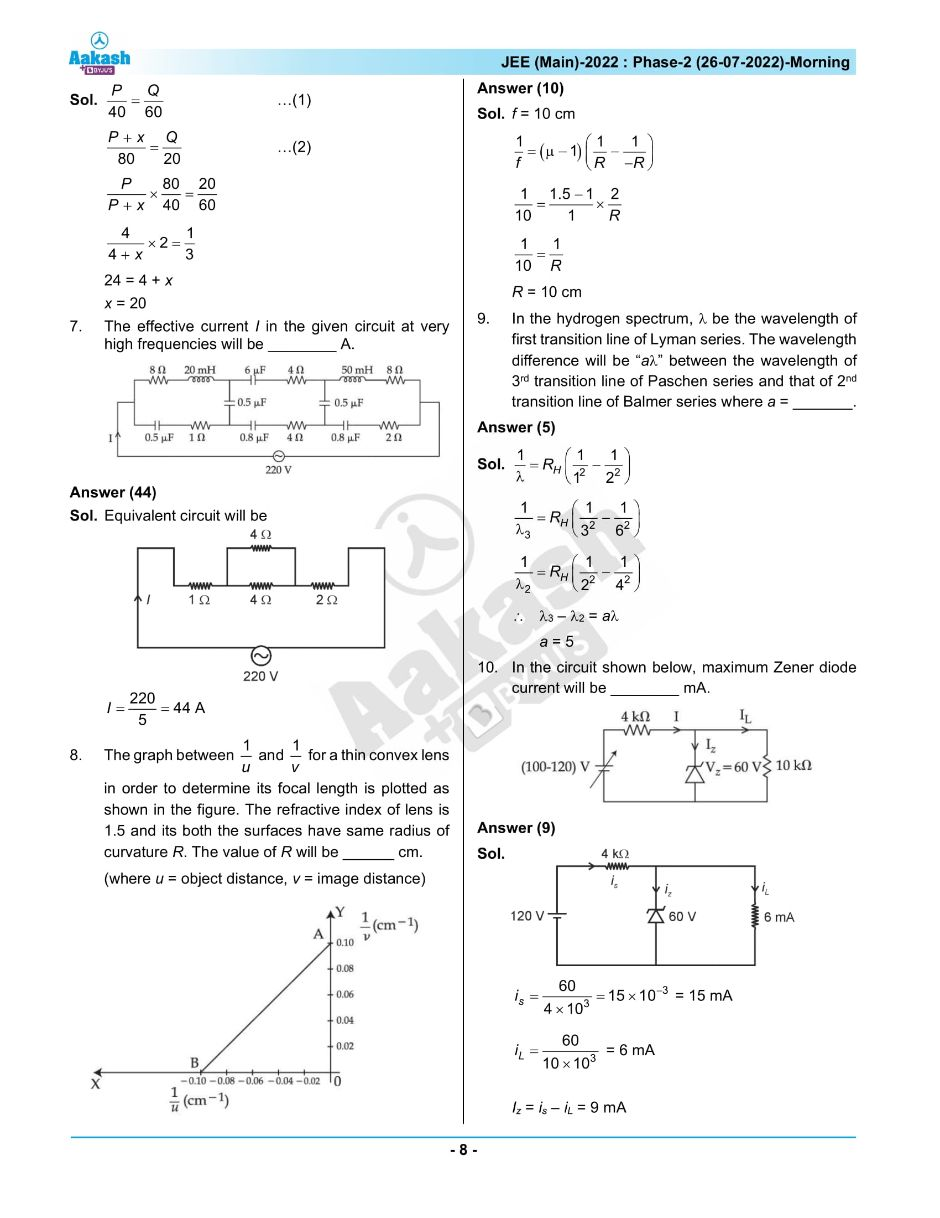## JEE Main 2022 July 26th Shift 1 Paper Analysis